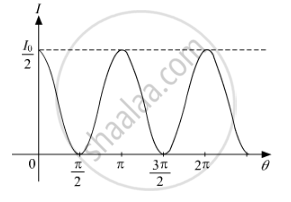Department of Pre-University Education, KarnatakaPUC Karnataka Science Class 12
Share

# Unpolarised light is passed through a polaroid P1. When this polarised beam passes through another polaroid P2 and if the pass axis of P2 makes angle θ with the pass axis of P1 - Physics

#### Question

Unpolarised light is passed through a polaroid P1. When this polarised beam passes through another polaroid P2 and if the pass axis of P2 makes angle θ with the pass axis of P1, then write the expression for the polarised beam passing through P2. Draw a plot showing the variation of intensity when θ varies from 0 to 2π.

#### Solution

When unpolarised light passes through the polariser P1 the intensity drops to half that is I_o/2 and when this polarised light passes through the polariser P2 the instensity of the emitted light is

I=I_0/2cos^2 theta

(θ is the angle between polariser P1 and P2)

The instensity distrubution is as shown below:Is there an error in this question or solution?